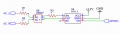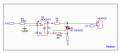# ESP32 - right dimensioning of zero cross detection circuit

#### angel0ne

Joined Sep 14, 2023
3
I need to detect the zero cross of AC main (220V - 50Hz) with an ESP32 to make a phase shift control of a resistive load. Searching the web I found this circuit but I have some doubts on how to dimensions the resistor values properly.
Can you please tell me if my reasoning is right or wrong?In order to calculate the values of R1 and R2 I made this calculations:
$V_{peak}=220*\sqrt{2} \simeq 312V \\ I_f=\frac{312V}{220 k\Omega} = 1,41 mA \\ P_{R} = V_{peak}*I_f = 312V * 1,41 mA \simeq 440 mW$
I want to limit the forward current to the diode in the bridge rectifier and also the power dissipation through the input resistors (R1 and R2).
With the same approach R3 should limit the current into the anode of the photodiode inside the H11L1:
$I_F^{max} = 30mA \\ R_3 = \frac{V_{peak}}{10mA} = \frac{312V}{10mA} = 31,2\Omega \simeq 33\Omega \\ P_{R_3} = 312 * 10mA = 3,120 W$
This is the first doubt that I have: Shouldn't the current be limited to 1.41mA because of R1 and R2?
I'm asking this because 3W of power is huge for a single resistor.
If you wondering why I'm using H11L1 instead a common optocoupler (e.g 4N35), thats because I need a "clean" square wave output to use ESP32 interrupt on raising edge. ESP32 is too fast for the AC frequency and if you use a 4N35 you'll get a lot of false positive triggering. Arduino, that is much slower than ESP32, doesn't have that issue.

Lets move to the phase control circuit to dim a 220V heater:R5 is 1K because the $I_{TSM}^{max}$ of the Triac in the MOC3021 is 1A, with $R_5=1K\Omega \to I_{TSM} = \frac{312V}{1K\Omega} = 312 mA$

Do I also have to limit the current that flows into the BT139's gate? If not why?

#### angel0ne

Joined Sep 14, 2023
3
Is there anyone that could help?

#### Ian0

Joined Aug 7, 2020
8,947
There's no good reason to use phase control for a heater. It just generates a whole load of interference.
Proportional control switches the load on for whole mains cycles so it doesn't generate interference. You can then use a MOC3041 opto-triac, and you don't need a zero-crossing detector.
To use proportional control, switch the MOC3041 with a low frequency PWM, say a period of 200ms, with the on time proportional to the power you need.

•Alec_t

#### angel0ne

Joined Sep 14, 2023
3
There's no good reason to use phase control for a heater. It just generates a whole load of interference.
Proportional control switches the load on for whole mains cycles so it doesn't generate interference. You can then use a MOC3041 opto-triac, and you don't need a zero-crossing detector.
To use proportional control, switch the MOC3041 with a low frequency PWM, say a period of 200ms, with the on time proportional to the power you need.
Thanks for the reply. Using an opto-triac with zero-cross detection feature (like the MOC3041) can be driven with a PWM signal? I'm asking this because everyone who I asked told me that I need phase control to drive an AC load with a PWM signal.

Just to better understand how power dissipation works, can you please tell me if the componente dimensioning of my circuite is correct or not? Can I use a SMD resistors with 220V?

Thanks

#### Ian0

Joined Aug 7, 2020
8,947
Thanks for the reply. Using an opto-triac with zero-cross detection feature (like the MOC3041) can be driven with a PWM signal? I'm asking this because everyone who I asked told me that I need phase control to drive an AC load with a PWM signal.

Just to better understand how power dissipation works, can you please tell me if the componente dimensioning of my circuite is correct or not? Can I use a SMD resistors with 220V?

Thanks
Surface mount resistors tend to be rated at 200V, so you need two in series for 230V AC.
The 2515 size will be ok for mains, but two 1206s tend to be cheaper.
You can use a low value for the triac gate resistor. All you need to do is keep the opto-triac current below its maximum.
The power dissipated in the gate resistor is negligible because it only conducts for about 1us before the main triac conducts and shorts it out.

Triacs are usually equipped with built in resistance between G and MT1: I have never found additional resistors between G and MT1 to be necessary, unless you have a triac with a very sensitive gate. If you are triggering with an opto-triac, use 50mA gate triacs - they are more robust that sensitive gate types.

Only use phase control if you absolutely must - in circumstances where you need a fast response such as filament lamps (remember those?) and universal motors. If you do, you will not pass EMC regulations without an LC filter.
Otherwise, where there is a large thermal mass (more than can easily be heated up in 20ms) use proportional control.

•angel0ne